A* Search Algorithm

• Difficulty Level : Hard
• Last Updated : 24 Dec, 2021

Motivation
To approximate the shortest path in real-life situations, like- in maps, games where there can be many hindrances.
We can consider a 2D Grid having several obstacles and we start from a source cell (colored red below) to reach towards a goal cell (colored green below)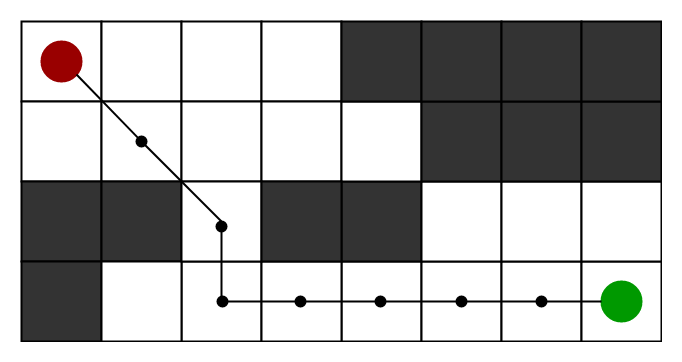What is A* Search Algorithm?
A* Search algorithm is one of the best and popular technique used in path-finding and graph traversals.

Why A* Search Algorithm?
Informally speaking, A* Search algorithms, unlike other traversal techniques, it has “brains”. What it means is that it is really a smart algorithm which separates it from the other conventional algorithms. This fact is cleared in detail in below sections.
And it is also worth mentioning that many games and web-based maps use this algorithm to find the shortest path very efficiently (approximation).

Explanation
Consider a square grid having many obstacles and we are given a starting cell and a target cell. We want to reach the target cell (if possible) from the starting cell as quickly as possible. Here A* Search Algorithm comes to the rescue.
What A* Search Algorithm does is that at each step it picks the node according to a value-‘f’ which is a parameter equal to the sum of two other parameters – ‘g’ and ‘h’. At each step it picks the node/cell having the lowest ‘f’, and process that node/cell.
We define ‘g’ and ‘h’ as simply as possible below
g = the movement cost to move from the starting point to a given square on the grid, following the path generated to get there.
h = the estimated movement cost to move from that given square on the grid to the final destination. This is often referred to as the heuristic, which is nothing but a kind of smart guess. We really don’t know the actual distance until we find the path, because all sorts of things can be in the way (walls, water, etc.). There can be many ways to calculate this ‘h’ which are discussed in the later sections.

Algorithm
We create two lists – Open List and Closed List (just like Dijkstra Algorithm)

// A* Search Algorithm
1.  Initialize the open list
2.  Initialize the closed list
put the starting node on the open
list (you can leave its f at zero)

3.  while the open list is not empty
a) find the node with the least f on
the open list, call it "q"

b) pop q off the open list

c) generate q's 8 successors and set their
parents to q

d) for each successor
i) if successor is the goal, stop search
successor.g = q.g + distance between
successor and q
successor.h = distance from goal to
successor (This can be done using many
ways, we will discuss three heuristics-
Manhattan, Diagonal and Euclidean
Heuristics)

successor.f = successor.g + successor.h

ii) if a node with the same position as
successor is in the OPEN list which has a
lower f than successor, skip this successor

iii) if a node with the same position as
successor  is in the CLOSED list which has
a lower f than successor, skip this successor
otherwise, add  the node to the open list
end (for loop)

e) push q on the closed list
end (while loop)

So suppose as in the below figure if we want to reach the target cell from the source cell, then the A* Search algorithm would follow path as shown below. Note that the below figure is made by considering Euclidean Distance as a heuristics.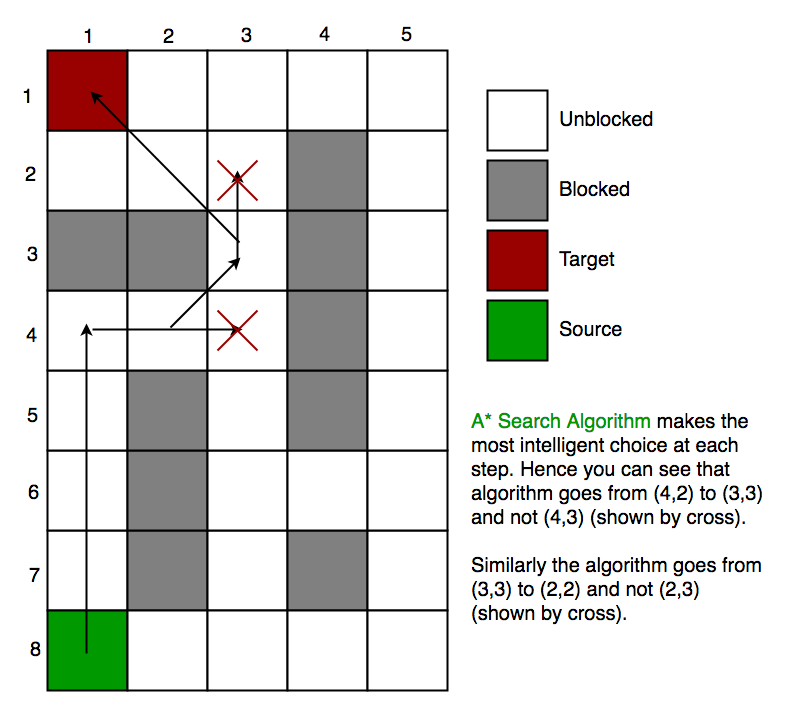Heuristics
We can calculate g but how to calculate h ?
We can do things.
A) Either calculate the exact value of h (which is certainly time consuming).
OR
B ) Approximate the value of h using some heuristics (less time consuming).
We will discuss both of the methods.
A) Exact Heuristics
We can find exact values of h, but that is generally very time consuming.
Below are some of the methods to calculate the exact value of h.
1) Pre-compute the distance between each pair of cells before running the A* Search Algorithm.
2) If there are no blocked cells/obstacles then we can just find the exact value of h without any pre-computation using the distance formula/Euclidean Distance

B) Approximation Heuristics –
There are generally three approximation heuristics to calculate h –

1) Manhattan Distance –

• It is nothing but the sum of absolute values of differences in the goal’s x and y coordinates and the current cell’s x and y coordinates respectively, i.e.,
h = abs (current_cell.x – goal.x) +
abs (current_cell.y – goal.y)
• When to use this heuristic? – When we are allowed to move only in four directions only (right, left, top, bottom)

The Manhattan Distance Heuristics is shown by the below figure (assume red spot as source cell and green spot as target cell).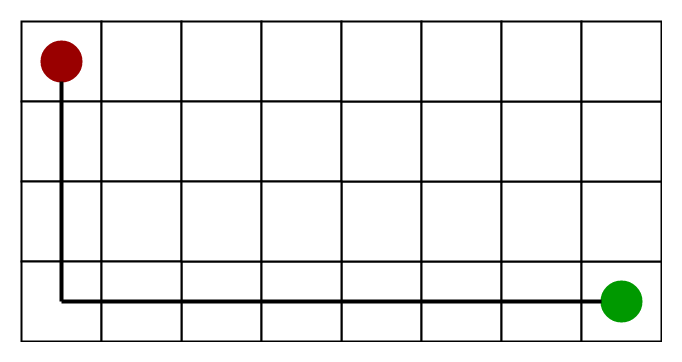2) Diagonal Distance-

• It is nothing but the maximum of absolute values of differences in the goal’s x and y coordinates and the current cell’s x and y coordinates respectively, i.e.,
dx = abs(current_cell.x – goal.x)
dy = abs(current_cell.y – goal.y)

h = D * (dx + dy) + (D2 - 2 * D) * min(dx, dy)

where D is length of each node(usually = 1) and D2 is diagonal distance between each node (usually = sqrt(2) ).
• When to use this heuristic? – When we are allowed to move in eight directions only (similar to a move of a King in Chess)

The Diagonal Distance Heuristics is shown by the below figure (assume red spot as source cell and green spot as target cell).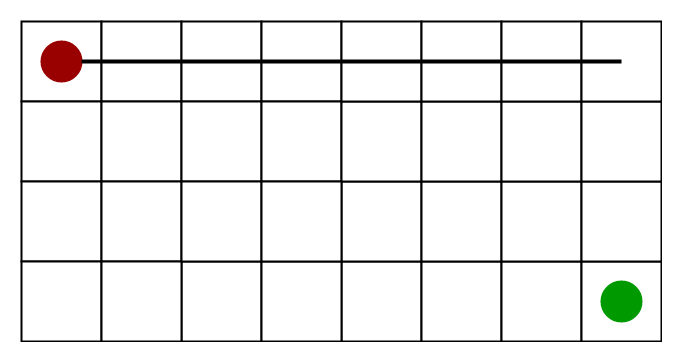3) Euclidean Distance-

• As it is clear from its name, it is nothing but the distance between the current cell and the goal cell using the distance formula
h = sqrt ( (current_cell.x – goal.x)2 +
(current_cell.y – goal.y)2 )
• When to use this heuristic? – When we are allowed to move in any directions.

The Euclidean Distance Heuristics is shown by the below figure (assume red spot as source cell and green spot as target cell).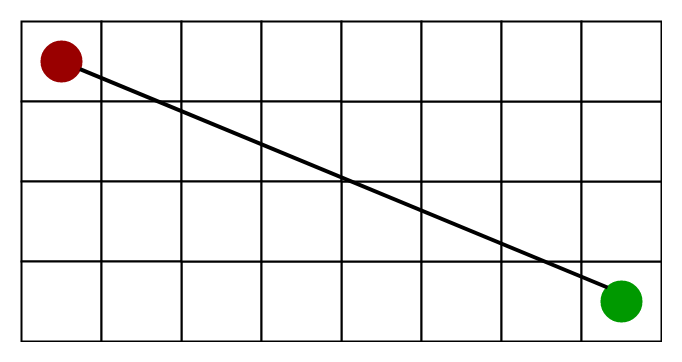Relation (Similarity and Differences) with other algorithms-
Dijkstra is a special case of A* Search Algorithm, where h = 0 for all nodes.

Implementation
We can use any data structure to implement open list and closed list but for best performance, we use a set data structure of C++ STL(implemented as Red-Black Tree) and a boolean hash table for a closed list.
The implementations are similar to Dijkstra’s algorithm. If we use a Fibonacci heap to implement the open list instead of a binary heap/self-balancing tree, then the performance will become better (as Fibonacci heap takes O(1) average time to insert into open list and to decrease key)

Also to reduce the time taken to calculate g, we will use dynamic programming.

C++14

Limitations
Although being the best pathfinding algorithm around, A* Search Algorithm doesn’t produce the shortest path always, as it relies heavily on heuristics / approximations to calculate – h

Applications
This is the most interesting part of A* Search Algorithm. They are used in games! But how?
Ever played Tower Defense Games
Tower defense is a type of strategy video game where the goal is to defend a player’s territories or possessions by obstructing enemy attackers, usually achieved by placing defensive structures on or along their path of attack.
A* Search Algorithm is often used to find the shortest path from one point to another point. You can use this for each enemy to find a path to the goal.
One example of this is the very popular game- Warcraft III

What if the search space is not a grid and is a graph ?
The same rules applies there also. The example of grid is taken for the simplicity of understanding. So we can find the shortest path between the source node and the target node in a graph using this A* Search Algorithm, just like we did for a 2D Grid.

Time Complexity
Considering a graph, it may take us to travel all the edge to reach the destination cell from the source cell [For example, consider a graph where source and destination nodes are connected by a series of edges, like – 0(source) –>1 –> 2 –> 3 (target)
So the worse case time complexity is O(E), where E is the number of edges in the graph

Auxiliary Space In the worse case we can have all the edges inside the open list, so required auxiliary space in worst case is O(V), where V is the total number of vertices.

Ever wondered how to make a game like- Pacman where there are many such obstacles. Can we use A* Search Algorithm to find the correct way ?
Think about it as a fun exercise.

In our program, the obstacles are fixed. What if the obstacles are moving ? Interested readers may see here an excellent discussion on this topic.

Summary
So when to use DFS over A*, when to use Dijkstra over A* to find the shortest paths ?
We can summarise this as below-
1) One source and One Destination-
→ Use A* Search Algorithm (For Unweighted as well as Weighted Graphs)
2) One Source, All Destination –
→ Use BFS (For Unweighted Graphs)
→ Use Dijkstra (For Weighted Graphs without negative weights)
→ Use Bellman Ford (For Weighted Graphs with negative weights)
3) Between every pair of nodes-
→ Floyd-Warshall
→ Johnson’s Algorithm

Related Article:
Best First Search (Informed Search)

This article is contributed by Rachit Belwariar. If you like GeeksforGeeks and would like to contribute, you can also write an article and mail your article to review-team@geeksforgeeks.org. See your article appearing on the GeeksforGeeks main page and help other Geeks.